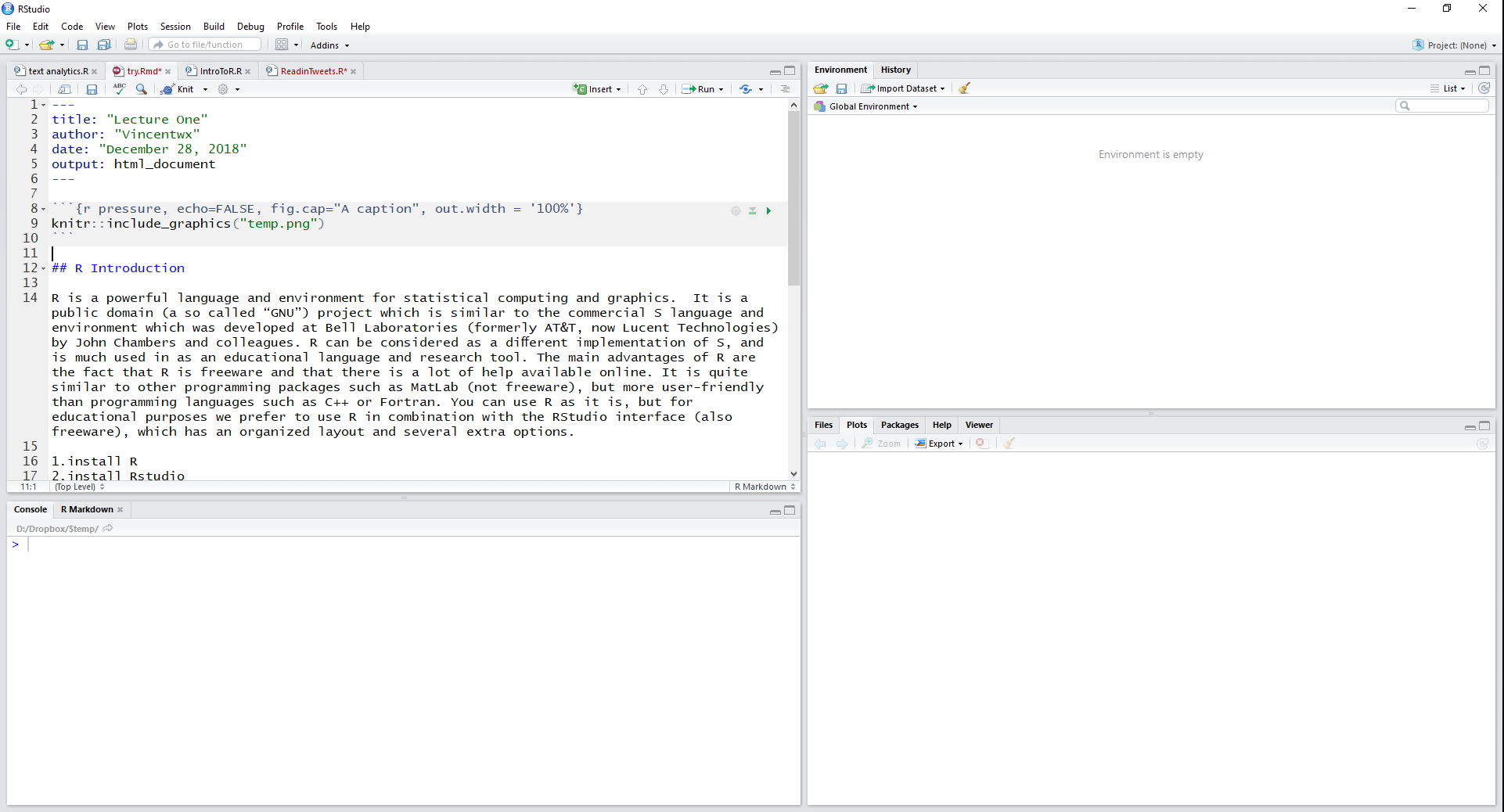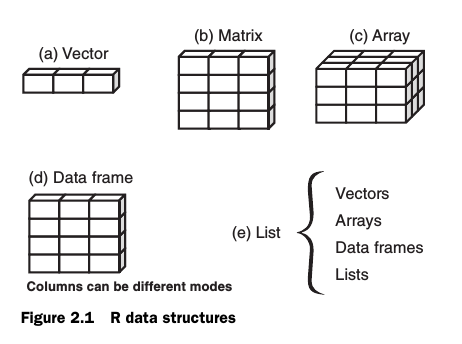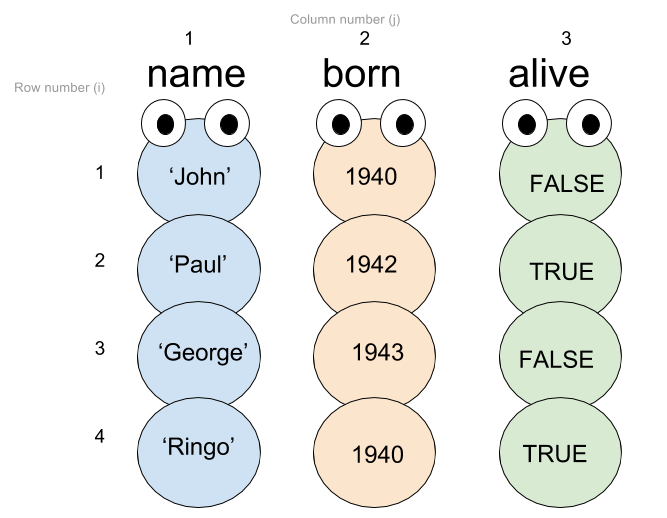# R Introduction

R is a powerful language and environment for statistical computing and graphics. It is a public domain (a so called “GNU”) project which is similar to the commercial S language and environment which was develo-ped at Bell Laboratories (formerly AT&T, now Lucent Technologies) by John Chambers and colleagues. R can be considered as a diﬀerent implementation of S, and is much used in as an educational language and research tool. The main advantages of R are the fact that R is freeware and that there is a lot of help available online. It is quite similar to other programming packages such as MatLab (not freeware), but more user-friendly than programming languages such as C++ or Fortran. You can use R as it is, but for educational purposes we prefer to use R in combination with the RStudio interface (also freeware), which has an organized layout and several extra options.

Getting started
1. install R.
2. install Rstudio.
3. R layout: console window | editor window | environment/history window | ﬁles/plots/packages/help window.Rstudio Layout

### 1. Set working directory

Before you start working, please set your working directory to where all your data and script ﬁles are or should be stored.

setwd("D:/temp")

or manually choose directory (be mind of the pop-out window)

setwd(choose.dir())

R can do many statistical and data analyses. They are organized in so-called packages or libraries. With the standard installation, most common packages are installed.

install.packages("wordcloud") #Install the package
library(wordcloud) #load the package before use it

### 3. R basics

Some ﬁrst examples of R command

print("hello world") #how to run the commend line (click Run OR control+Enter)
10^2 + 36 #calculations
sqrt(9) #square root
3 < 4 #logic expression
2 + 2 == 4 #double-equal sign for equal in mathematic expressions

### 4. R environment

you can save values in a variable

x = 85 # OR x <- 85
#<- or = means assign in R

You can see that “x”" appears in the workspace window, which means that R now save the value in “x”

x #require x, show value in x
x*5 # do calculations with x
x = x +5 #assign x with new value

exercise: calculate bmi

height = 1.75
weight = 60
bmi = weight/height^2 #^caret
bmi
##  19.59184

check whether your bmi fall into normal range

bmi > 18.5 & bmi < 25 #range of normal weight
##  TRUE
bmi < 18.5 | bmi > 25 #RANGE FOR NOT SO GOOD value
##  FALSE

### 5. Functions

You call a function by typing its name, followed by one or more arguments to that function Let’s try using the sum function

sum(1,2,4,7) #sum() is a function
rep("penny",times=3) #times=3 is arguement specifying the function rep

the most useful function in R

help(rep)

### 6. Data structures

vector,matrix,and data frame
* vector: a list of values, also called arrays, 1-dimensional; A vector’s values can be numbers, strings, logical values, as long as they’re all the same type.
* Matrix: 2-dimensional data structure including rows and column.
* data.frame: same format with matrix, the difference columns in data frame can be different data types.
* list: a container, can be mixture of data structureSource: Kabacoff (2011) R in Action

6.1. Vector

x = c() #the c function combine Values into a Vector or List
x = c(1,2,4,7)
x #request x
sum(x)
y = c("a","b","c","d") #array of characters
y #access the second value in y
y[c(2,3)] #access multiple values
y = "cat" #assign new value to the third value in y
y[4:5] = c("dog","bear") #assign new value to y
#Now try to access the 2nd, 4th, and 5th words
y[c(2,4,5)] # not y[2,4,5]

6.2. matrix
Matrices are nothing more than 2-dimensional vectors. To deﬁne a matrix, use the function matrix:

m = matrix()
m = matrix(0,3,4)

#use a vector to initialize a matrix's value, and transform it into a 3 by 4 matrix
x = 1:12
m = matrix(x,3,4) #transform vector into matrix

#Try getting a value from the matrix:
m[2,3]

#assign with new value
m[2,3] = 0

#get an entire row of the matrix:
m[2,]

#OR the entire column:
m[,3]

m[,3:4]
m[c(1,3),]

6.3. data frame
data frame is a data set that includes multiple types of data, such as numeric and string. A data frame is a matrix with variable names above the columns, visually, it looks like this:Source:Github/chainsawriot/jmsc6041_extras

df = data.frame()
#manually input three vectors
age = c(20,25,30)
gender = c("male","female","male")
score = c(65,75,85)

# create new data frame using function 'data.frame'
df = data.frame(age,gender,score)

#data frame subsetting:
df[1,2]
#using $to request certain column by name names(df) #request all the variable names in df df$gender

# add a new variable named 'midterm' to the data frame
df$midterm = c(7,8,9) #create a new variable based on existing variables in the data frame df$sum = df$score + df$midterm

6.4.list
list is a container that can contain all types of data, include lists:

L = list(v1=x,v2=y,matrix=m,dataframe=df)
L #show values in ls
L[] #request values in list
L$v1 #request values by calling name ### 7. Class check the type of values in the data or variable. An value in R can have several types of ‘class’. The most important three are ‘numeric’, ‘character’ and datetime. You can ask R what class a certain variable is by typing class(). class(20) #numeric ##  "numeric" class("male") #character ##  "character" d = Sys.Date() class(d) #Date ##  "Date" ### 8. Programming tools 8.1. if statement #use the bmi example again bmi = 19 if (bmi> 18.5 & bmi < 25) { print("your bmi is normal") } else { print("not normal") } 8.2. for loops h = seq(from=1, to=8) s = NULL for(i in 1:length(h)){ s[i] = h[i] * 10 } s # Data Management 1. export data data(mtcars) #load the defalt dataset 'mtcatrs' in R write.csv(mtcars,"mtcars.csv",row.names = FALSE) #row.names=FALSE, means dont write row names #prefer csv, can be easily edit and read by other software write.table(mtcars,"mtcars.txt",row.names = FALSE) #export data in .txt 2. import csv file mydata = read.csv("mtcars.csv",header=TRUE) #header=TRUE, means the first row is header mydata = read.table("mtcars.txt",header=TRUE,sep=",") 3. rename columns in data frame names(mydata) names(mydata) = "fuel_economy" 4. recode data # recode the engine displacement into three categories: low, medium, high mydata$rank[mydata$disp <= 160] = "L" mydata$rank[mydata$disp > 160 & mydata$disp <= 300] = "M"
mydata$rank[mydata$disp > 300] = "H"
mydata$rank 5. sunsetting dataset #Selecting/keeping variables newdata1 = mydata[,c(1,3)] #keep the first and third columns newdata1 = mydata[,c("fuel economy","rank")] #keep the first and third columns # Dropping variables newdata2 = mydata[,c(-2:-5)] #drop the second to the fifth column in the dataframe #Selecting observations mydata[mydata$rank == "H",] #select rows which rank equal to 'H'
mydata[mydata$wt > 4,] #select rows which wt larger than 4 6. deal with misssing value mydata$cyl = NA #assign a NA to the dataset, NA means missing value
sum(mydata$cyl) #reture NA, because there is a missing value in the vector sum(mydata$cyl,na.rm=TRUE) #na.rm means remove missing value equal to TRUE

which(is.na(mydata$cyl))# identify the NA values 7. inspect data head(mydata) #showing the first 6 rows str(mydata) #Display the Structure of the data summary(mydata) #descirptives of the data class(mydata$cyl) #data class
table(mydata\$cyl) #frequency

You are refer to this cheat sheet for all the R basics https://www.rstudio.com/wp-content/uploads/2016/10/r-cheat-sheet-3.pdf